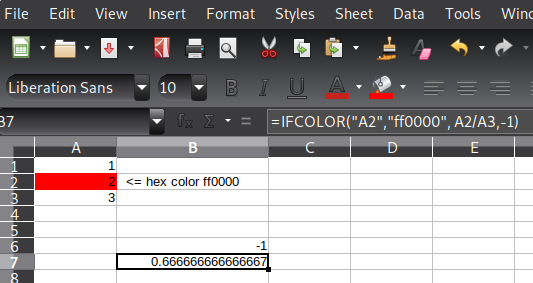# Do math only if the cell has particular color?

Hello,

I would like to know if it is possible to have a formula similar to this :

=IF(A1=#FF0000,F1/E1,"")

If A1 = Red, do the math, otherwise the cell is empty. I’ve tried scouring the forum for an answer and couldn’t find anything similar.

Thank you

Hello,

the following macro function (see end of this post) does what you want. Just put it into Tools → Macros → “Edit Macros” and you can call it from inside any cell via

``````=IFCOLOR("A2","ff0000", A2/A3,-1)
``````
• The 1st argument is the cell to check quoted as a string
• The 2nd argument is a hexadecimal color value quoted as a string To view or set the hex value of a cell open the color picker ref1. ref2..
• the 3rd argument is the result to set when the background color matches
• the 4th argument is the result to set when the background color deos not match

Example Screenshot:Here the macro function you have to import:

``````Function IFCOLOR(cellName As String, hexColor As String, ifValue as variant, elseValue as variant)

REM remove trailing spaces
cellName = Trim(cellName)
hexColor = UCase(Trim(hexColor))

Dim decColor As Long

REM check length
if (Len(hexColor) <> 6) then
SUMIFCOLOR = "ERR: invalid hex code, length <> 6"
Exit Function
endif

REM check chars
For i = 1 To Len(hexColor)
c = Mid(hexColor, i, 1)
Select Case c
Case "0","1","2","3","4","5","6","7","8","9","A","B","C","D","E","F"
Case Else
SUMIFCOLOR = "ERR: invalid hex code, invalid hex char"
Exit Function
End Select
Next

REM convert to long
decColor = CLng("&H" & hexColor)  REM convert to long

REM sum result
sum = 0.0

Set oSheet = ThisComponent.CurrentController.ActiveSheet
Set oCell = oSheet.getCellRangeByName(cellName)

if(oCell.CellBackColor = decColor) then
IFCOLOR = ifValue
else
IFCOLOR = elseValue
endif

End Function
``````

Hope that helps.

To show the community your question has been answered, click the ✓ next to the correct answer, and “upvote” by clicking on the ^ arrow of any helpful answers. These are the mechanisms for communicating the quality of the Q&A on this site. Thanks!

nice workthe OP request may be questionable … don’t get used to use such things … but as it came up … having a solution is fine,

(deccolor is a cruel attribute, hex would be easier)

The hex value can be converted using the DECIMAL() function, like `DECIMAL("C9211E";16)`

Nice. I did not know about the DECIMAL() function.
I’ll update the answer to make it more userfriendly. In the end ii went with the the HEX2DEC function, becasue one less argument. - In my personal opinion calling createUnoService for one function … make the whole solution a bit bloated but … as long as it works.

Just as a side note, HEX2DEC() does not accept an optional “0x…” prefix which DECIMAL(…;16) does; I meant that rather as a user-provided argument conversion than being part of the macro function.

However, the bloated callFunction() call is unnecessary as BASIC has a CLng() function to convert a hex string if it is prefixed with “&H”, so this instead of ofa.callFunction() should work:

``````decColor = CLng("&H" & hexColor)
``````

(untested in the context of the macro function).

I keep learning new stuff. Thanks.
I’ll test your suggestion and update the snippet when it works.

[Update 1] This looks much nicer with the CLng() function. I also added some crude checks to give quick error feedback. I might add some checks for the range argument later. Improvement suggestions are very much welcome.

[Update 2] i changed the macro a bit to make it a more direct answer for the question. It now has a very similar call syntax to the one requested in the question. Also i moved the previous macro function here How to sum() only cells where certain color is set, because the question is a better fit for it.

Hello,

no - this is not possible with standard functionality. All calculations are done using values of cells and not with attributes of cells (and the background / text color is an attribute of a cell).

PS I’m purposely not talking about user code (macros) and defining a function, which might provide values(!) of attributes. And I can’t resist to say, that the idea itself is questionable. If you format a cell a certain way, I’d assume you have some condition to do so. Hence try to use the same condition, which forces the formatting, in your `IF` statement.